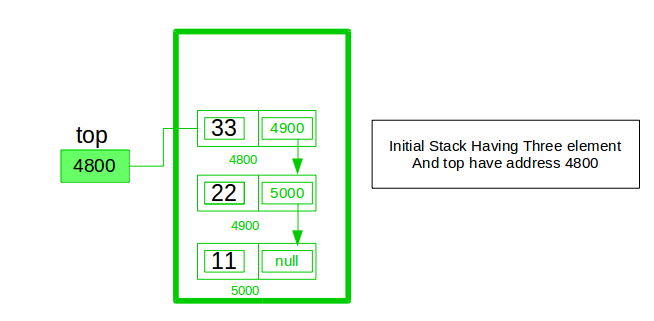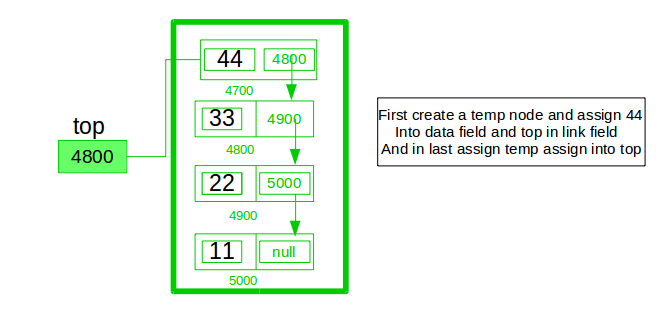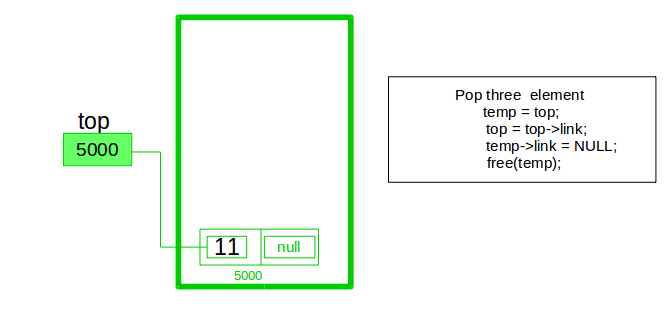Open in App
Not now

# Implement a stack using singly linked list

• Difficulty Level : Easy
• Last Updated : 11 Jan, 2023

To implement a stack using the singly linked list concept, all the singly linked list operations should be performed based on Stack operations LIFO(last in first out) and with the help of that knowledge, we are going to implement a stack using a singly linked list.

So we need to follow a simple rule in the implementation of a stack which is last in first out and all the operations can be performed with the help of a top variable. Let us learn how to perform Pop, Push, Peek, and Display operations in the following article:In the stack Implementation, a stack contains a top pointer. which is the “head” of the stack where pushing and popping items happens at the head of the list. The first node has a null in the link field and second node-link has the first node address in the link field and so on and the last node address is in the “top” pointer.

The main advantage of using a linked list over arrays is that it is possible to implement a stack that can shrink or grow as much as needed. Using an array will put a restriction on the maximum capacity of the array which can lead to stack overflow. Here each new node will be dynamically allocated. so overflow is not possible.

## Stack Operations:

• push(): Insert a new element into the stack i.e just insert a new element at the beginning of the linked list.
• pop(): Return the top element of the Stack i.e simply delete the first element from the linked list.
• peek(): Return the top element.
• display(): Print all elements in Stack.

## Push Operation:

• Initialise a node
• Update the value of that node by data i.e. node->data = data
• Now link this node to the top of the linked list
• And update top pointer to the current node

## Pop Operation:

• First Check whether there is any node present in the linked list or not, if not then return
• Otherwise make pointer let say temp to the top node and move forward the top node by 1 step
• Now free this temp node

## Peek Operation:

• Check if there is any node present or not, if not then return.
• Otherwise return the value of top node of the linked list

## Display Operation:

• Take a temp node and initialize it with top pointer
• Now start traversing temp till it encounters NULL
• Simultaneously print the value of the temp node

Below is the implementation of the above operations

## C++

 `// C++ program to Implement a stack ` `// using singly linked list ` `#include ` `using` `namespace` `std; ` ` `  `// creating a linked list; ` `class` `Node { ` `public``: ` `    ``int` `data; ` `    ``Node* link; ` `   `  `    ``// Constructor ` `    ``Node(``int` `n) ` `    ``{ ` `        ``this``->data = n; ` `        ``this``->link = NULL; ` `    ``} ` `}; ` ` `  `class` `Stack { ` `    ``Node* top; ` ` `  `public``: ` `    ``Stack() { top = NULL; } ` ` `  `    ``void` `push(``int` `data) ` `    ``{ ` ` `  `        ``// Create new node temp and allocate memory in heap ` `        ``Node* temp = ``new` `Node(data); ` ` `  `        ``// Check if stack (heap) is full. ` `        ``// Then inserting an element would ` `        ``// lead to stack overflow ` `        ``if` `(!temp) { ` `            ``cout << ``"\nStack Overflow"``; ` `            ``exit``(1); ` `        ``} ` ` `  `        ``// Initialize data into temp data field ` `        ``temp->data = data; ` ` `  `        ``// Put top pointer reference into temp link ` `        ``temp->link = top; ` ` `  `        ``// Make temp as top of Stack ` `        ``top = temp; ` `    ``} ` ` `  `    ``// Utility function to check if ` `    ``// the stack is empty or not ` `    ``bool` `isEmpty() ` `    ``{ ` `        ``// If top is NULL it means that ` `        ``// there are no elements are in stack ` `        ``return` `top == NULL; ` `    ``} ` ` `  `    ``// Utility function to return top element in a stack ` `    ``int` `peek() ` `    ``{ ` `        ``// If stack is not empty , return the top element ` `        ``if` `(!isEmpty()) ` `            ``return` `top->data; ` `        ``else` `            ``exit``(1); ` `    ``} ` ` `  `    ``// Function to remove ` `    ``// a key from given queue q ` `    ``void` `pop() ` `    ``{ ` `        ``Node* temp; ` ` `  `        ``// Check for stack underflow ` `        ``if` `(top == NULL) { ` `            ``cout << ``"\nStack Underflow"` `<< endl; ` `            ``exit``(1); ` `        ``} ` `        ``else` `{ ` ` `  `            ``// Assign top to temp ` `            ``temp = top; ` ` `  `            ``// Assign second node to top ` `            ``top = top->link; ` ` `  `            ``// This will automatically destroy ` `            ``// the link between first node and second node ` ` `  `            ``// Release memory of top node ` `            ``// i.e delete the node ` `            ``free``(temp); ` `        ``} ` `    ``} ` ` `  `    ``// Function to print all the ` `    ``// elements of the stack ` `    ``void` `display() ` `    ``{ ` `        ``Node* temp; ` ` `  `        ``// Check for stack underflow ` `        ``if` `(top == NULL) { ` `            ``cout << ``"\nStack Underflow"``; ` `            ``exit``(1); ` `        ``} ` `        ``else` `{ ` `            ``temp = top; ` `            ``while` `(temp != NULL) { ` ` `  `                ``// Print node data ` `                ``cout << temp->data; ` ` `  `                ``// Assign temp link to temp ` `                ``temp = temp->link; ` `                ``if` `(temp != NULL) ` `                    ``cout << ``" -> "``; ` `            ``} ` `        ``} ` `    ``} ` `}; ` ` `  `// Driven Program ` `int` `main() ` `{ ` `    ``// Creating a stack ` `    ``Stack s; ` ` `  `    ``// Push the elements of stack ` `    ``s.push(11); ` `    ``s.push(22); ` `    ``s.push(33); ` `    ``s.push(44); ` ` `  `    ``// Display stack elements ` `    ``s.display(); ` ` `  `    ``// Print top element of stack ` `    ``cout << ``"\nTop element is "` `<< s.peek() << endl; ` ` `  `    ``// Delete top elements of stack ` `    ``s.pop(); ` `    ``s.pop(); ` ` `  `    ``// Display stack elements ` `    ``s.display(); ` ` `  `    ``// Print top element of stack ` `    ``cout << ``"\nTop element is "` `<< s.peek() << endl; ` ` `  `    ``return` `0; ` `}`

## Java

 `// Java program to Implement a stack ` `// using singly linked list ` `// import package ` `import` `static` `java.lang.System.exit; ` ` `  `// Driver code ` `class` `GFG { ` `    ``public` `static` `void` `main(String[] args) ` `    ``{ ` `        ``// create Object of Implementing class ` `        ``StackUsingLinkedlist obj ` `            ``= ``new` `StackUsingLinkedlist(); ` `        ``// insert Stack value ` `        ``obj.push(``11``); ` `        ``obj.push(``22``); ` `        ``obj.push(``33``); ` `        ``obj.push(``44``); ` ` `  `        ``// print Stack elements ` `        ``obj.display(); ` ` `  `        ``// print Top element of Stack ` `        ``System.out.printf(``"\nTop element is %d\n"``, ` `                          ``obj.peek()); ` ` `  `        ``// Delete top element of Stack ` `        ``obj.pop(); ` `        ``obj.pop(); ` ` `  `        ``// print Stack elements ` `        ``obj.display(); ` ` `  `        ``// print Top element of Stack ` `        ``System.out.printf(``"\nTop element is %d\n"``, ` `                          ``obj.peek()); ` `    ``} ` `} ` ` `  `// Create Stack Using Linked list ` `class` `StackUsingLinkedlist { ` ` `  `    ``// A linked list node ` `    ``private` `class` `Node { ` ` `  `        ``int` `data; ``// integer data ` `        ``Node link; ``// reference variable Node type ` `    ``} ` `    ``// create global top reference variable global ` `    ``Node top; ` `    ``// Constructor ` `    ``StackUsingLinkedlist() { ``this``.top = ``null``; } ` ` `  `    ``// Utility function to add an element x in the stack ` `    ``public` `void` `push(``int` `x) ``// insert at the beginning ` `    ``{ ` `        ``// create new node temp and allocate memory ` `        ``Node temp = ``new` `Node(); ` ` `  `        ``// check if stack (heap) is full. Then inserting an ` `        ``//  element would lead to stack overflow ` `        ``if` `(temp == ``null``) { ` `            ``System.out.print(``"\nHeap Overflow"``); ` `            ``return``; ` `        ``} ` ` `  `        ``// initialize data into temp data field ` `        ``temp.data = x; ` ` `  `        ``// put top reference into temp link ` `        ``temp.link = top; ` ` `  `        ``// update top reference ` `        ``top = temp; ` `    ``} ` ` `  `    ``// Utility function to check if the stack is empty or ` `    ``// not ` `    ``public` `boolean` `isEmpty() { ``return` `top == ``null``; } ` ` `  `    ``// Utility function to return top element in a stack ` `    ``public` `int` `peek() ` `    ``{ ` `        ``// check for empty stack ` `        ``if` `(!isEmpty()) { ` `            ``return` `top.data; ` `        ``} ` `        ``else` `{ ` `            ``System.out.println(``"Stack is empty"``); ` `            ``return` `-``1``; ` `        ``} ` `    ``} ` ` `  `    ``// Utility function to pop top element from the stack ` `    ``public` `void` `pop() ``// remove at the beginning ` `    ``{ ` `        ``// check for stack underflow ` `        ``if` `(top == ``null``) { ` `            ``System.out.print(``"\nStack Underflow"``); ` `            ``return``; ` `        ``} ` ` `  `        ``// update the top pointer to point to the next node ` `        ``top = (top).link; ` `    ``} ` ` `  `    ``public` `void` `display() ` `    ``{ ` `        ``// check for stack underflow ` `        ``if` `(top == ``null``) { ` `            ``System.out.printf(``"\nStack Underflow"``); ` `            ``exit(``1``); ` `        ``} ` `        ``else` `{ ` `            ``Node temp = top; ` `            ``while` `(temp != ``null``) { ` ` `  `                ``// print node data ` `                ``System.out.print(temp.data); ` ` `  `                ``// assign temp link to temp ` `                ``temp = temp.link; ` `                ``if``(temp != ``null``) ` `                    ``System.out.print(``" -> "``); ` `            ``} ` `        ``} ` `    ``} ` `}`

## Python3

 `# python3 program to Implement a stack ` `# using singly linked list ` ` `  `class` `Node: ` ` `  `    ``# Class to create nodes of linked list ` `    ``# constructor initializes node automatically ` `    ``def` `__init__(``self``, data): ` `        ``self``.data ``=` `data ` `        ``self``.``next` `=` `None` ` `  ` `  `class` `Stack: ` ` `  `    ``# head is default NULL ` `    ``def` `__init__(``self``): ` `        ``self``.head ``=` `None` ` `  `    ``# Checks if stack is empty ` `    ``def` `isempty(``self``): ` `        ``if` `self``.head ``=``=` `None``: ` `            ``return` `True` `        ``else``: ` `            ``return` `False` ` `  `    ``# Method to add data to the stack ` `    ``# adds to the start of the stack ` `    ``def` `push(``self``, data): ` ` `  `        ``if` `self``.head ``=``=` `None``: ` `            ``self``.head ``=` `Node(data) ` ` `  `        ``else``: ` `            ``newnode ``=` `Node(data) ` `            ``newnode.``next` `=` `self``.head ` `            ``self``.head ``=` `newnode ` ` `  `    ``# Remove element that is the current head (start of the stack) ` `    ``def` `pop(``self``): ` ` `  `        ``if` `self``.isempty(): ` `            ``return` `None` ` `  `        ``else``: ` `            ``# Removes the head node and makes ` `            ``# the preceding one the new head ` `            ``poppednode ``=` `self``.head ` `            ``self``.head ``=` `self``.head.``next` `            ``poppednode.``next` `=` `None` `            ``return` `poppednode.data ` ` `  `    ``# Returns the head node data ` `    ``def` `peek(``self``): ` ` `  `        ``if` `self``.isempty(): ` `            ``return` `None` ` `  `        ``else``: ` `            ``return` `self``.head.data ` ` `  `    ``# Prints out the stack ` `    ``def` `display(``self``): ` ` `  `        ``iternode ``=` `self``.head ` `        ``if` `self``.isempty(): ` `            ``print``(``"Stack Underflow"``) ` ` `  `        ``else``: ` ` `  `            ``while``(iternode !``=` `None``): ` ` `  `                ``print``(iternode.data, end ``=` `"") ` `                ``iternode ``=` `iternode.``next` `                ``if``(iternode !``=` `None``): ` `                    ``print``(``" -> "``, end ``=` `"") ` `            ``return` ` `  ` `  `# Driver code ` `if` `__name__ ``=``=` `"__main__"``: ` `  ``MyStack ``=` `Stack() ` `   `  `  ``MyStack.push(``11``) ` `  ``MyStack.push(``22``) ` `  ``MyStack.push(``33``) ` `  ``MyStack.push(``44``) ` ` `  `  ``# Display stack elements ` `  ``MyStack.display() ` ` `  `  ``# Print top element of stack ` `  ``print``(``"\nTop element is "``, MyStack.peek()) ` ` `  `  ``# Delete top elements of stack ` `  ``MyStack.pop() ` `  ``MyStack.pop() ` ` `  `  ``# Display stack elements ` `  ``MyStack.display() ` ` `  `  ``# Print top element of stack ` `  ``print``(``"\nTop element is "``, MyStack.peek()) ` ` `  `# This code is contributed by Mathew George `

## C#

 `// C# program to Implement a stack ` `// using singly linked list ` ` `  `// import package ` `using` `System; ` ` `  `// Create Stack Using Linked list ` `public` `class` `StackUsingLinkedlist { ` ` `  `    ``// A linked list node ` `    ``private` `class` `Node { ` `        ``// integer data ` `        ``public` `int` `data; ` ` `  `        ``// reference variable Node type ` `        ``public` `Node link; ` `    ``} ` ` `  `    ``// create global top reference variable ` `    ``Node top; ` ` `  `    ``// Constructor ` `    ``public` `StackUsingLinkedlist() { ``this``.top = ``null``; } ` ` `  `    ``// Utility function to add ` `    ``// an element x in the stack ` `    ``// insert at the beginning ` `    ``public` `void` `push(``int` `x) ` `    ``{ ` `        ``// create new node temp and allocate memory ` `        ``Node temp = ``new` `Node(); ` ` `  `        ``// check if stack (heap) is full. ` `        ``// Then inserting an element ` `        ``// would lead to stack overflow ` `        ``if` `(temp == ``null``) { ` `            ``Console.Write(``"\nHeap Overflow"``); ` `            ``return``; ` `        ``} ` ` `  `        ``// initialize data into temp data field ` `        ``temp.data = x; ` ` `  `        ``// put top reference into temp link ` `        ``temp.link = top; ` ` `  `        ``// update top reference ` `        ``top = temp; ` `    ``} ` ` `  `    ``// Utility function to check if ` `    ``// the stack is empty or not ` `    ``public` `bool` `isEmpty() { ``return` `top == ``null``; } ` ` `  `    ``// Utility function to return ` `    ``// top element in a stack ` `    ``public` `int` `peek() ` `    ``{ ` `        ``// check for empty stack ` `        ``if` `(!isEmpty()) { ` `            ``return` `top.data; ` `        ``} ` `        ``else` `{ ` `            ``Console.WriteLine(``"Stack is empty"``); ` `            ``return` `-1; ` `        ``} ` `    ``} ` ` `  `    ``// Utility function to pop top element from the stack ` `    ``public` `void` `pop() ``// remove at the beginning ` `    ``{ ` `        ``// check for stack underflow ` `        ``if` `(top == ``null``) { ` `            ``Console.Write(``"\nStack Underflow"``); ` `            ``return``; ` `        ``} ` ` `  `        ``// update the top pointer to ` `        ``// point to the next node ` `        ``top = (top).link; ` `    ``} ` ` `  `    ``public` `void` `display() ` `    ``{ ` `        ``// check for stack underflow ` `        ``if` `(top == ``null``) { ` `            ``Console.Write(``"\nStack Underflow"``); ` `            ``return``; ` `        ``} ` `        ``else` `{ ` `            ``Node temp = top; ` `            ``while` `(temp != ``null``) { ` ` `  `                ``// print node data ` `                ``Console.Write(temp.data); ` ` `  `                ``// assign temp link to temp ` `                ``temp = temp.link; ` `                ``if``(temp != ``null``) ` `                    ``Console.Write(``" -> "``); ` `            ``} ` `        ``} ` `    ``} ` `} ` ` `  `// Driver code ` `public` `class` `GFG { ` `    ``public` `static` `void` `Main(String[] args) ` `    ``{ ` `        ``// create Object of Implementing class ` `        ``StackUsingLinkedlist obj ` `            ``= ``new` `StackUsingLinkedlist(); ` ` `  `        ``// insert Stack value ` `        ``obj.push(11); ` `        ``obj.push(22); ` `        ``obj.push(33); ` `        ``obj.push(44); ` ` `  `        ``// print Stack elements ` `        ``obj.display(); ` ` `  `        ``// print Top element of Stack ` `        ``Console.Write(``"\nTop element is {0}\n"``, obj.peek()); ` ` `  `        ``// Delete top element of Stack ` `        ``obj.pop(); ` `        ``obj.pop(); ` ` `  `        ``// print Stack elements ` `        ``obj.display(); ` ` `  `        ``// print Top element of Stack ` `        ``Console.Write(``"\nTop element is {0}\n"``, obj.peek()); ` `    ``} ` `} ` ` `  `// This code is contributed by 29AjayKumar`

## Javascript

 `// Javascript program to Implement a stack ` `// using singly linked list ` `// import package ` ` `  `// A linked list node ` `class Node ` `{ ` `    ``constructor() ` `    ``{ ` `        ``this``.data=0; ` `        ``this``.link=``null``; ` `    ``} ` `} ` ` `  `// Create Stack Using Linked list ` `class StackUsingLinkedlist ` `{ ` `    ``constructor() ` `    ``{ ` `        ``this``.top=``null``; ` `    ``} ` `     `  `    ``// Utility function to add an element x in the stack ` `    ``push(x) ` `    ``{ ` `        ``// create new node temp and allocate memory ` `        ``let temp = ``new` `Node(); ` `  `  `        ``// check if stack (heap) is full. Then inserting an ` `        ``//  element would lead to stack overflow ` `        ``if` `(temp == ``null``) { ` `            ``document.write(``"
Heap Overflow"``); ` `            ``return``; ` `        ``} ` `  `  `        ``// initialize data into temp data field ` `        ``temp.data = x; ` `  `  `        ``// put top reference into temp link ` `        ``temp.link = ``this``.top; ` `  `  `        ``// update top reference ` `        ``this``.top = temp; ` `    ``} ` `     `  `     ``// Utility function to check if the stack is empty or not ` `    ``isEmpty() ` `    ``{ ` `         ``return` `this``.top == ``null``; ` `    ``} ` `     `  `    ``// Utility function to return top element in a stack     ` `    ``peek() ` `    ``{ ` `        ``// check for empty stack ` `        ``if` `(!``this``.isEmpty()) { ` `            ``return` `this``.top.data; ` `        ``} ` `        ``else` `{ ` `            ``document.write(``"Stack is empty
"``); ` `            ``return` `-1; ` `        ``} ` `    ``} ` `     `  `    ``// Utility function to pop top element from the stack ` `    ``pop() ``// remove at the beginning ` `    ``{ ` `        ``// check for stack underflow ` `        ``if` `(``this``.top == ``null``) { ` `            ``document.write(``"
Stack Underflow"``); ` `            ``return``; ` `        ``} ` `  `  `        ``// update the top pointer to point to the next node ` `        ``this``.top = ``this``.top.link; ` `    ``} ` `     `  `    ``display() ` `    ``{ ` `        ``// check for stack underflow ` `        ``if` `(``this``.top == ``null``) { ` `            ``document.write(``"
Stack Underflow"``); ` `             `  `        ``} ` `        ``else` `{ ` `            ``let temp = ``this``.top; ` `            ``while` `(temp != ``null``) { ` `  `  `                ``// print node data ` `                ``document.write(temp.data+``"->"``); ` `  `  `                ``// assign temp link to temp ` `                ``temp = temp.link; ` `            ``} ` `        ``} ` `    ``} ` `} ` ` `  `// main class ` ` `  `// create Object of Implementing class ` `let obj = ``new` `StackUsingLinkedlist(); ` `// insert Stack value ` `obj.push(11); ` `obj.push(22); ` `obj.push(33); ` `obj.push(44); ` ` `  `// print Stack elements ` `obj.display(); ` ` `  `// print Top element of Stack ` `document.write(``"
Top element is "``, obj.peek()+``"
"``); ` ` `  `// Delete top element of Stack ` `obj.pop(); ` `obj.pop(); ` ` `  `// print Stack elements ` `obj.display(); ` ` `  `// print Top element of Stack ` `document.write(``"
Top element is "``, obj.peek()+``"
"``); ` ` `  `// This code is contributed by rag2127 `

Output

```44 -> 33 -> 22 -> 11
Top element is 44
22 -> 11
Top element is 22```

Time Complexity: O(1), for all push(), pop(), and peek(), as we are not performing any kind of traversal over the list. We perform all the operations through the current pointer only.
Auxiliary Space: O(N), where N is the size of the stack

My Personal Notes arrow_drop_up
Related Articles